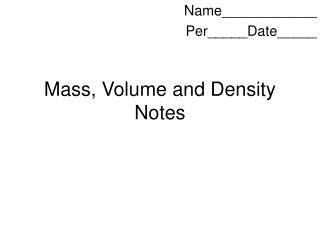DownloadDownload PresentationMass, Volume and Density Notes

# Mass, Volume and Density Notes

Télécharger la présentation## Mass, Volume and Density Notes

- - - - - - - - - - - - - - - - - - - - - - - - - - - E N D - - - - - - - - - - - - - - - - - - - - - - - - - - -
##### Presentation Transcript

1. Mass, Volume and Density Notes Name____________ Per_____Date_____

2. Metric System • Developed by the French in the late 1700’s. • Based on powers of ten, so it is very easy to use. • Used by almost every country in the world, with the notable exception of the USA. • Especially used by scientists. • Abbreviated SI, which is French for Systeme International.

3. Mass • Mass is the amount of matter that makes up an object. • A golf ball and a ping pong ball are the same size, but the golf ball has a lot more matter in it. So the golf ball will have more mass. • The SI unit for mass is the gram. • A paper clip has a mass of about one gram. • The mass of an object will not change unless we add or subtract matter from it.

4. Measuring Mass • We will use a triple beam balance and a digital top-loading scale to measure mass.

5. Weight and Mass • Notice that Jill’s mass never changes. Jill is 30kg of little girl no matter where she goes!

6. Volume • Volume is the amount of space an object consumes. • The base unit for volume is the liter. • There are two methods for finding volume.

7. Volume of Regular Objects • We can find the volume of box shapes with the formula Volume = length x width x height. • In this case the units would be cubic centimeters (cm3). • So a box 2 cm x 3 cm x 5cm would have a volume of 30 cm3 2 cm 5 cm 3 cm

8. Volume of Irregular Objects We measure volume of irregular objects with a graduated cylinder. This method is called water displacement Liquids form a curved surface in graduated cylinders. Take your reading at the low point of the curve or meniscus

9. Water Displacement • Pour 7 ml of water in a graduated cylinder. If a rock causes the level to rise to 9 ml, the rock must have a volume of 2 ml.

10. Liquid Volume • 1 cm3 = 1 ml • 1 cm3 of water = 1 ml of water = 1 gram of water

11. Water Mass and Volume • 50 ml (50cm3) of water would have a mass of 50 grams • 1 Liter of water would have a mass of _______? • 1 L = 1000 mL so its mass would be 1000 grams or a kilogram.

12. Density • Density is the amount of matter (mass) compared to the amount of space (volume) the object occupies.

13. Density • Density = mass/volume • Remember, all fractions are division problems. • Since the unit for mass is grams, and the unit for volume is ml or cm3, then the unit for density is g/ml, or g/ cm3

14. Density Formula Wheel • Formula wheels make it easy to solve density problems. • Cover the property you are trying to find, and do what is left over. • To find density, cover the word density. You have mass over volume remaining. So divide mass by volume to find density! Mass Density Volume

15. Understanding Density • In the following illustration, each square will represent 1 cm3. • Each g will represent 1 gram. • Mass = 24g • Volume = 8 cm3 • Density = ?g/cm3